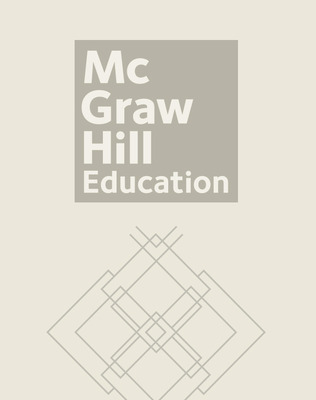# Number Sense 2003

Contemporary's popular Number Sense encourages students to explore number relationships to build a concrete understanding of mathematical operations, problem-solving strategies, and real-life applications, using a highly visual and active approach to introduce basic mathematics concepts.Each book in the Number Sense series illustrates a basic math skill with a step-by-step approach, followed with lots of practice. Lessons are carefully designed to simultaneously build students' mastery and self-confidence.

10 Student books each cover a particular a particular concept. The Teacher's Resource Guide and Answer Key provides a placement test, additional activities, answer keys, and CASAS correlation.

Sort by: